# Definition of Fourier Transform

The Fourier theorem states that any waveform can be duplicated by the superposition of a series of sine and cosine waves. As an example, the following Fourier expansion of sine waves provides an approximation of a square wave.

The three curves in the plot show the first one term (black line), four terms (blue line), and sixteen terms (red line) in the Fourier expansion. As more terms are added the superposition of sine waves better matches a square wave.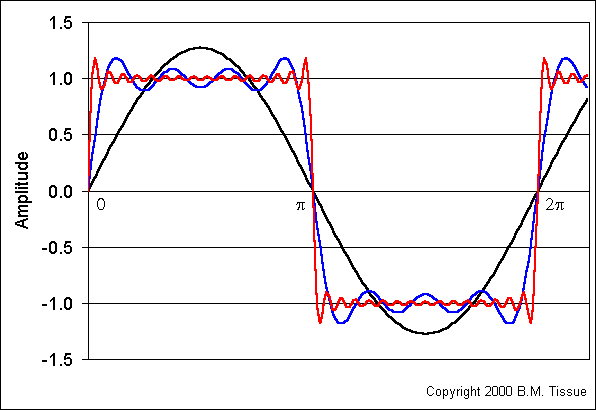The Fourier Transform uses the above concept to convert between two different descriptions of a physical system.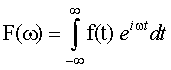In these equations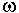is angular frequency (2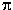x frequency), t is time, and: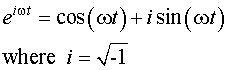The F() function gives the frequencies at which the signal is non-zero and the f(t) function gives the times at which the signal is non-zero. Both of these functions are suitable descriptions of a waveform or physical system.

Given a function in time, f(t), we can transform it to an equivalent fuction in frequency, F(). We can look at the second expression in detail to understand what is happening.To do the transform we multiply f(t) times [cos(t)+i sin(t)]. We do this at all times between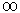and -. DFT and FFT

Experimental data usually consists of discrete data points rather than a continuous function as used in the equations above. The Discrete Fourier-Transform (DFT) is an algorithm for doing the transform with discrete data. The DFT is an order N2 calculation, meaning that the number of multiplications is equal to the square of the number of data points. This algorithm has been supplanted by Fast Fourier-Transform (FFT) algorithms, which reduce redundancies and take much less computer time. The order of this calculation is NlogN.

A key experimental parameter is the sampling frequency, which must be at least twice as large as the highest frequency component that is present in the data. This sampling rate is called the Nyquist critical frequency. Sampling at less than the Nyquist frequency results in aliasing of the result. Aliasing can be prevented by filtering out all frequencies above the Nyquist frequency so that they do not create artifacts in the transformed spectrum. The Fourier-Transform in Analytical Instrumentation

Fast Fourier-Transform (FFT) algorithms are used in NMR, infrared, Raman, and mass spectrometry instrumentation. The advantages are:

The multiplex advantage arises from recording all signal frequencies simultaneously. The throughput advantage arises because no physical slit is necessary to obtain resolution in the resulting spectra.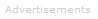Search the Dictionary for More Terms